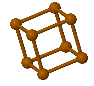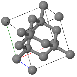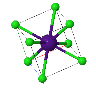Menu Outline Exercise Questions Appendices Lectures Student Projects Books Sections Introduction Atoms Molecules Crystal Structure Crystal Diffraction Crystal Binding Photons Phonons Electrons Band Model Crystal Physics Semiconductors

PHY.K02UF Molecular and Solid State Physics

## Miller indices

### The translation vector

Every point of a Bravais lattice can be reached from the origin by a translation vector of the form,

$\begin{equation} \vec{T}_{hkl} = h\vec{a}_1 + k\vec{a}_2 + l\vec{a}_3, \end{equation}$

where $\vec{a}_1$, $\vec{a}_2$, $\vec{a}_3$ are the primitive lattice vectors and $h$, $k$, and $l$ are integers called the Miller indices. The direction that the vector $\vec{T}_{hkl}$ points is denoted with square brackets, $[hkl]$. If there are directions that are equivalent due to symmetry any of the equivalent directions are specified by angular brackets <$hkl$>.

For example, in a cubic system:
<100> = , , , [-100], [0-10], [00-1]
<110> = , [-1-10], [1-10], [-110], , [-10-1], [-101], [10-1], , [0-1-1], [0-11], [01-1].

Often the minus signs are drawn as overlines [-10-1] = $\left[\overline{1}0\overline{1}\right]$. This is pronounced one-bar zero one-bar.

### Planes

Using Miller indices, planes are denoted with curved brackets $(hkl)$. The $(hkl)$ plane intercepts the axis in the direction of $\vec{a}_1$ at $\frac{|\vec{a}_1|}{h}$, the axis in the direction of $\vec{a}_2$ at $\frac{|\vec{a}_2|}{k}$, and the axis in the direction of $\vec{a}_3$ at $\frac{|\vec{a}_3|}{l}$.

Equivalent planes are denoted with curly brackets $\{hkl\}$. Three points that can be used to define the $(hkl)$ plane are $\left( \frac{a_{1x}}{h},\frac{a_{1y}}{h},\frac{a_{1z}}{h} \right)$, $\left(\frac{a_{2x}}{k},\frac{a_{2y}}{k},\frac{a_{2z}}{k} \right)$, and $\left(\frac{a_{3x}}{l},\frac{a_{3y}}{l},\frac{a_{3z}}{l}\right)$. The normal vector to this plane can be determined by taking the cross product of two vectors in the plane. If $(h,k,l \ne 0)$, two suitable vectors are $\vec{v}_1=\frac{\vec{a}_1}{h}-\frac{\vec{a}_3}{l}$ and $\vec{v}_2=\frac{\vec{a}_2}{k}-\frac{\vec{a}_3}{l}$. The unit vector normal to the $(hkl)$ plane is,

$$\hat{n}_{hkl}=\frac{\vec{v}_1\times \vec{v}_2}{|\vec{v}_1\times \vec{v}_2|}.$$

For cubic crystals, $\vec{T}_{hkl}$ is normal to $(hkl)$ and parallel to $\hat{n}_{hkl}$ but this is not generally true for crystals with other symmetries.

Primitive lattice vectors:

 $\vec{a}_1=$ $\hat{x}+$ $\hat{y}+$ $\hat{z}$ [m] $\vec{a}_2=$ $\hat{x}+$ $\hat{y}+$ $\hat{z}$ [m] $\vec{a}_3=$ $\hat{x}+$ $\hat{y}+$ $\hat{z}$ [m]

Miller indices:

 $h=$ $k=$ $l=$

Primitive unit cells:

Conventional unit cells:

JSmol can be used to draw crystalographic planes of different crystal structures. The JSmol models of some common crystal structures are linked below.Simple CubicFace Centered CubicBody Centered CubicHexagonal Close PackedDiamondNaClCsClZincblendeWurzitePerovskite